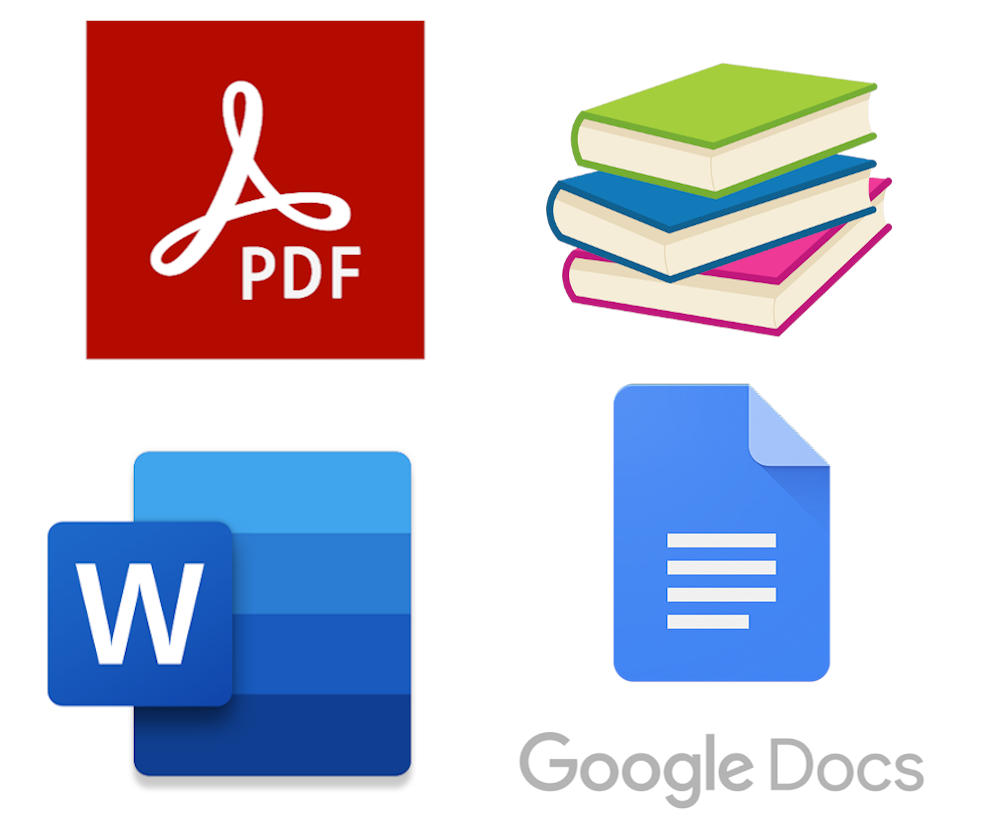# Give your students feedback,meaningfully and efficiently.

## Students Show Step-by-Step Work

Students can start with a blank Free Math document, copying down and working through problems just as they would in paper notebooks.

Students save their work as a file and submit it through an LMS in response to an assignment.

## Simultaneously Review All Assignments

Complete solutions are shown, grouped by similar final answer.

You can award partial credit and give feedback to students that need help.

You don't need to type in an answer key, Free Math just provides an organized view of all student work.Roland Smoker
Math Instructor
Conestoga Christian School

Free Math App is an excellent tool for seamlessly grading student work without timely preparation. Students are able access any mathematical symbol many word processing tools lack, enabling them to display their math work accurately and quickly.Jose
Math Teacher
Kidder County High School

I would just like to compliment you guys on the work you've been doing with the website. This has been a life saver. All of my classes are using this and some students have had to quarantine but they can still work on the HW and submit it.Krystina Wood
Mathematics Teacher
Toledo High School

Your site has made these difficult times of distance learning so much easier to manage as a teacher. I've raved about your site to several teachers and we're using it in all of our middle and high school classes.

## Use All of Your Existing Materials

You can assign problems from anywhere including a textbook, worksheet or digital document like a Google Doc, Microsoft Word file or a PDF.

The flexible grading experience means you don't need to convert your materials. Students just reference problems from anywhere and produce digital work that can be reviewed in Free Math immediately with no setup ahead of time.## Embrace Visual Learning

Students can add digital drawings and graphs to their assignments, or paste an image from another site like Desmos or Geogebra.

When writing on paper works better for some students, they can quickly snap a picture of their notebooks with their webcam.

Direct integration is provided for Google Drive and Classroom, including gradebook integration.

For other LMSes and cloud storage providers, assignments and grading sessions save directly from the browser to files in your downloads folder and from there they can be uploaded to any service you use for your class.## Office Hours

Have questions about how to get started with Free Math?
Want to talk with the team about a feature suggestion?
Interested in finding ways to contribute to Free Math?

Schedule a time to meet with our team using the link below.

## Get Involved

Free Math is open source, which means the source code of the site is available to view, modify and redistribute under the terms of the

Help us build our revolution, Free Math has already been used by tens of thousands of students and teachers to help improve feedback and discussion in their classes.

Report Bug or Request Feature
Source Code

Help us bring simple freeform digital math assignments to the world's classrooms.

## Great for Many Areas of Math

### Algebra

$\frac{1}{x-4}+\frac{2}{x^2-16}=\frac{3}{x+4}$
$\frac{1}{x-4}+\frac{2}{\left(x-4\right)\left(x+4\right)}=\frac{3}{x+4}$
$\frac{1}{x-4}\cdot\left(\frac{x+4}{x+4}\right)+\frac{2}{\left(x-4\right)\left(x+4\right)}=\frac{3}{x+4}\cdot\left(\frac{x-4}{x-4}\right)$
$\frac{1\left(x+4\right)}{\left(x-4\right)\left(x+4\right)}+\frac{2}{\left(x-4\right)\left(x+4\right)}=\frac{3\left(x-4\right)}{\left(x+4\right)\left(x-4\right)}$
$1\left(x+4\right)+2=3\left(x-4\right)$
$x+6=3x-12$
$x+18=3x$
$18=2x$
$9=x$

### Calculus

$\int x\ln xdx$
$u=\ln x$
$dv=xdx$
$du=\frac{1}{x}dx$
$v=\frac{x^2}{2}$
$\int x\ln sdx=\frac{x^2}{2}\ln x-\int\frac{x^2}{2}\cdot\frac{1}{x}dx$
$\frac{x^2}{2}\ln x-\frac{1}{2}\int xdx$
$\frac{x^2}{2}\ln x-\frac{1}{2}\left(\frac{x^2}{2}\right)+c$
$\frac{x^2}{2}\ln x-\frac{1}{4}x^2+c$

### Physics

$\text{A ball is thrown from 1 m above the ground.}$
$\text{It is given an initial velocity of 20 m/s}$
$\text{At an angle of 40 degrees above the horizontal}$
$\text{Find the maximum height reached}$
$\text{And velocity at that point}$
$x\left(t\right)=v\cos\left(\theta\right)t=20\cos\left(40\right)t=15.3t$
$y\left(t\right)=y_0+v\sin\left(\theta\right)t-\frac{9.8t^2}{2}$
$y\left(t\right)=1+20\sin\left(40\right)t-4.9t^2$
$y\left(t\right)=1+12.9t-4.9t^2$
$v_y\left(t\right)=v\sin\left(\theta\right)-9.8t$
$v_y\left(t\right)=12.9-9.8t$
$\max\ height\ at\ v_y\left(t\right)=0$
$12.9-9.8t=0$
$-9.8t=-12.9$
$t=\frac{-12.9}{-9.8}=1.3$
$y\left(1.3\right)=1+12.9\left(1.3\right)-4.9\left(1.3\right)^2$
$y\left(1.3\right)=9.5\ m$
$y\ component\ of\ velocity\ is\ 0\ at\ highest\ pt$
$total\ velocity\ =v_x=15.3\ \frac{m}{s}$
Free Math is free software: you can redistribute it and/or modify it under the terms of the GNU General Public License as published by the Free Software Foundation, either version 3 of the License, or (at your option) any later version. Free Math is distributed in the hope that it will be useful, but WITHOUT ANY WARRANTY; without even the implied warranty of MERCHANTABILITY or FITNESS FOR A PARTICULAR PURPOSE. See the GNU General Public License for more details. You should have received a copy of the GNU General Public License along with Free Math. If not, see <http://www.gnu.org/licenses/>.